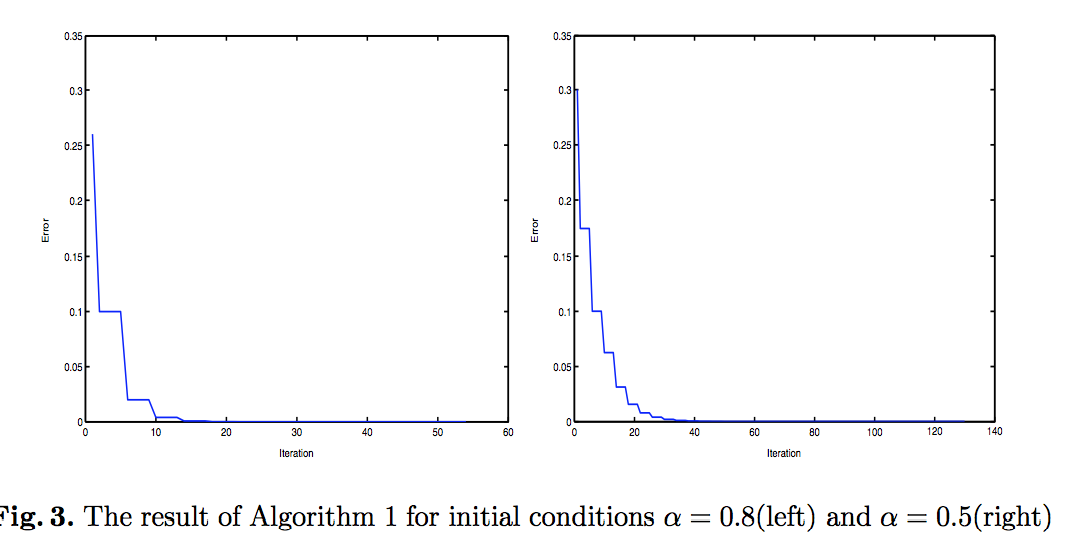# #54: New algorithms of neural fuzzy relation systems with min-implication composition, , and

Lecture Notes in Computer Science (Advances in Natural Computation), Volume 3612, pgs. 1132--1141, 2005

##### data mining, machine learning, classification

PlainText, Bibtex, PDF, DOI, Google Scholar

#### Abstract

Min-implication fuzzy relation equations based on Boolean- type implications can also be viewed as a way of implementing fuzzy associative memories with perfect recall. In this paper, fuzzy associative memories with perfect recall are constructed, and new on-line learning algorithms adapting the weights of its interconnections are incorporated into this neural network when the solution set of the fuzzy relation equa- tion is non-empty. These weight matrices are actually the least solution matrix and all maximal solution matrices of the fuzzy relation equation, respectively. The complete solution set of min-implication fuzzy relation equation can be determined by the maximal solution set of this equation.